Electron Gas Transverse Susceptibility

R. B. Laughlin May 19, 2008

(Submitted as coursework for Physics 373, Stanford University, Spring 2008)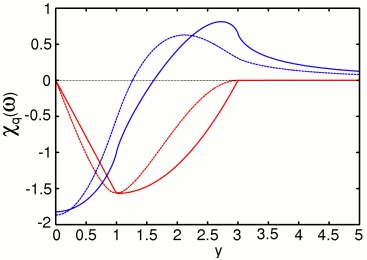Fig. 1: Comparison of the dimensionless part of the transverse current response function (dashed) with the dimensionless part of the density response (solid) for the case of x = 1. The blue and red curves are the real and imaginary parts, respectively. The computer code that generated this graph is available here. I plotted using gnuplot. The plot file is shown here.

The density-density response function of the electron gas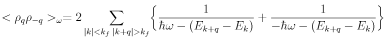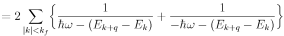where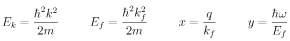has an expression in terms of simple functions of the form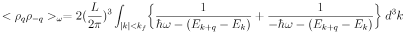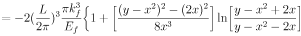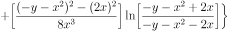The rule for the branch cuts is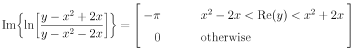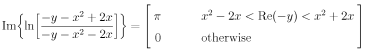The current-current response function on the other hand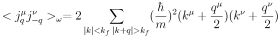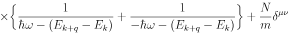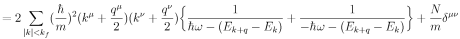cannot be expressed in terms of simple functions and so must be evaluated by computer. Actually, only the transverse part of the current response has this problem. Because of spherical symmetry, the response function decomposes into longitudinal and transverse parts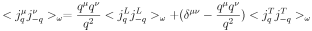The former is related to the density response by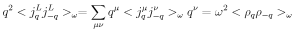by virtue of particle conservation (a.k.a. gauge invariance). The transverse part is given by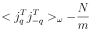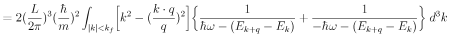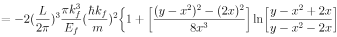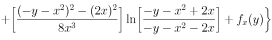where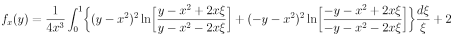This is a special function that looks worse than it actually is. You can generate it from its simple imaginary part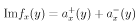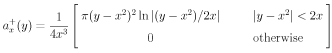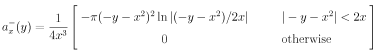by numerical hilbert transform. Moreover the effect of this function is rather benign. As can be seen from Fig. 1, it mainly smooths out the kinks of the density response.

The LaTeX source for the above equations is available here.